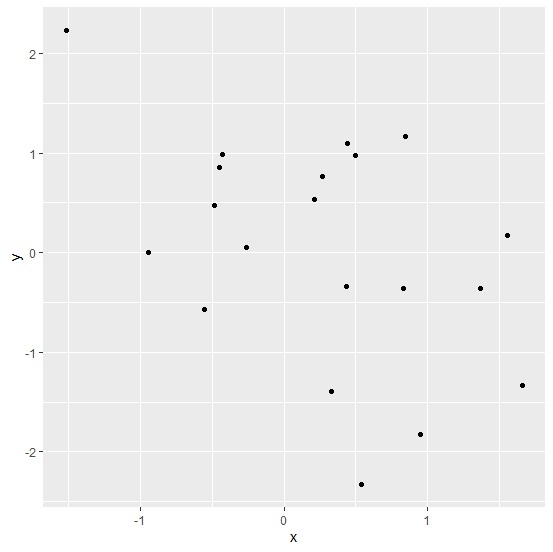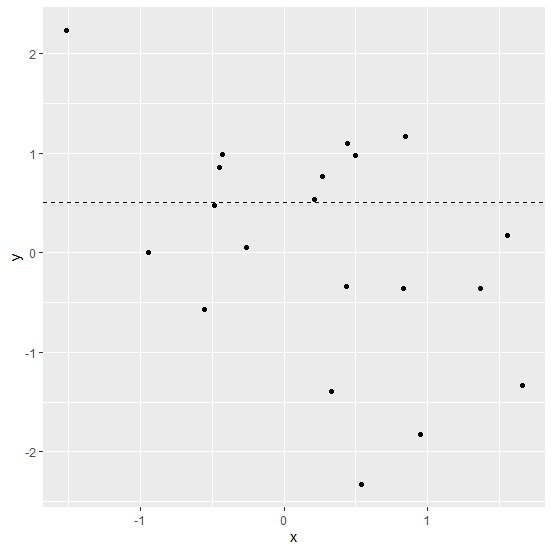# How to create a dashed horizontal line in a ggplot2 graph in R?

To create a dashed horizontal line in a ggplot2 graph in R, we can follow the below steps −

• First of all, create a data frame.
• Then, create a plot using ggplot2.
• After that, create the same plot with geom_hline function having horizontal line defined with y intercept and its type defined with line type argument.

## Create the data frame

Let's create a data frame as shown below −

Live Demo

> x<-rnorm(20)
> y<-rnorm(20)
> df<-data.frame(x,y)
> df

On executing, the above script generates the below output(this output will vary on your system due to randomization) −

         x       y
1 -0.2622735 0.050784727
2 -0.9453493 0.005828098
3 -0.5544653 -0.569278949
4   0.4988631 0.978485828
5   0.5389510 -2.328920035
6   0.4434926 1.099015564
7   0.2681379 0.760637085
8   1.5537351 0.172285069
9   0.9497421 -1.823161011
10 0.3323686 -1.394199992
11 0.2146744 0.538098034
12 0.8275667 -0.361978640
13 -0.4820211 0.477345035
14  0.4364038 -0.341711304
15 -0.4499373 0.854135140
16 1.6604468 -1.333167314
17 -0.4244539 0.989662861
18 1.3667020 -0.358490011
19 -1.5132316 2.234713443
20 0.8474354 1.162478362

## Create a plot using ggplot2

Let’s create a scatterplot between x and y −

> x<-rnorm(20)
> y<-rnorm(20)
> df<-data.frame(x,y)
> library(ggplot2)
> ggplot(df,aes(x,y))+geom_point()

## Output## Create the plot with dashed horizontal line

Using geom_hline to create the dashed horizontal line in the above plot with yintercept = 0.5 and linetype = 2 −

Live Demo

> x<-rnorm(20)
> y<-rnorm(20)
> df<-data.frame(x,y)
> library(ggplot2)
> ggplot(df,aes(x,y))+geom_point()+geom_hline(yintercept=0.5,linetype=2)

## Output